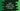# Swift program to check if two strings or characters are equal## String and Character equality in Swift 4 :

In swift, we can compare two strings or two characters using ’equal to’ and ’not equal to’ operators ( == and != ). Let’s explore how strings and characters are compared in swift with examples:

## Check if two strings and characters are equal or not :

We can use ’==’ or ’!=’ to check if two strings are equal or not :

``````import UIKit

let strOne = "Hello World !!"
let strTwo = "Hello World !!"

if strOne == strTwo {
print ("Both strings are equal")
}else{
print ("Strings are not equal")
}
``````

It will print :

``````Both strings are equal
``````

Similarly for characters :

``````import UIKit

let charOne = "A"
let charTwo = "A"

if charOne == charTwo {
print ("Both characters are equal")
}else{
print ("Characters are not equal")
}
``````

It will print the same :

``````Both characters are equal
``````

## How equality is determined :

Two String or Character values are equal only if their extended grapheme clusters are canonically equivalent.

In other words, in swift we can represent one character as a extended grapheme cluster which is one or more unicode scalar sequence. Even if two values are look same but they are not canonically equivalent, they will be considered as not equal.

i.e. é can be denoted as ”\u{E9}” or ”\u{65}\u{301}”. Both are canonically equivalent.

Similarly ’A’ can be represent as ”\u{41}” (english) or ”\u{410}” (Russian) . They are visually same but not canonically equal.

``````import UIKit

let charOne = "\u{E9}"
let charTwo = "\u{65}\u{301}"

let charThree = "\u{41}"
let charFour = "\u{410}"

print ("charOne ",charOne)
print ("charTwo ",charTwo)

if(charOne == charTwo){
print ("Above characters are equal ")
}else{
print ("Above characters are not equal")
}

print ("charThree ",charThree)
print ("charFour ",charFour)

if(charThree == charFour){
print ("Above characters are equal ")
}else{
print ("Above characters are not equal")
}
``````

It will give the following output :

``````charOne  é
charTwo  é
Above characters are equal
charThree  A
charFour  А
Above characters are not equal
``````